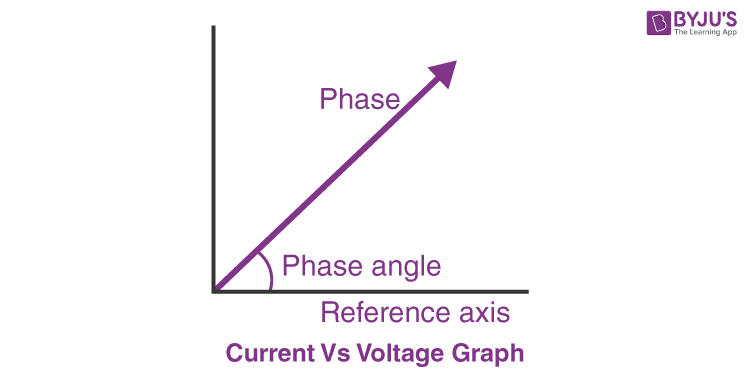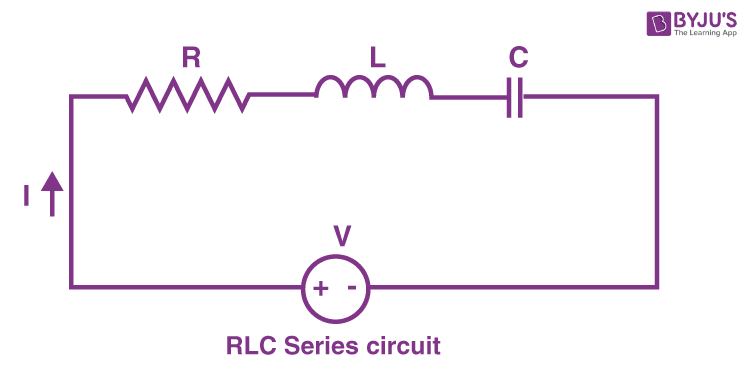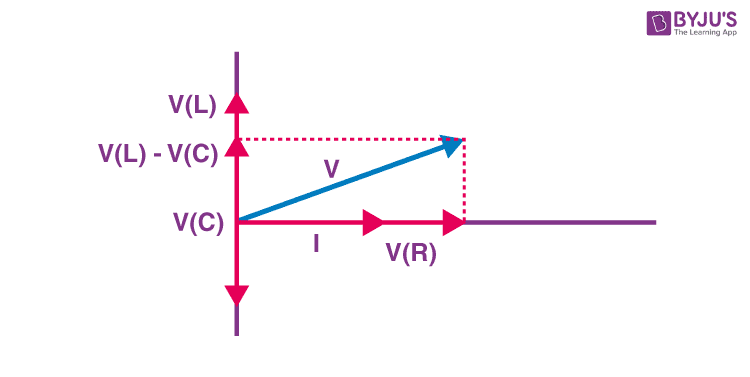# LCR Circuit : Analysis Of A LCR Series Circuit

In our article about the types of circuit, we discussed the two major types of circuit connection: Series and Parallel.

From the article, we understood that a series circuit is one in which the current remains the same along with each element. With this context, let us discuss the LCR circuit and its analysis in detail. An LCR circuit, also known as a resonant circuit, tuned circuit, or an RLC circuit, is an electrical circuit consisting of an inductor (L), capacitor (C) and resistor (R) connected in series or parallel. The LCR circuit analysis can be understood better in terms of phasors. A phasor is a rotating quantity.Current Vs Voltage Graph

For an inductor (L), if we consider I to be our reference axis, then voltage leads by 90°, and for the capacitor, the voltage lags by 90°. But the resistance, current and voltage phasors are always in phase.

Following is the table explaining other related concepts of the circuit:

## Analysis of An RLC Series Circuit

Let’s consider the following RLC circuit using the current across the circuit to be our reference phasor because it remains the same for all the components in a series RLC circuit.RLC Series circuit

As described above the overall phasor will look like below:Phasor diagram of current Vs voltage for resistor, inductor and capacitor for RLC series circuit

From the above phasor diagram we know that,

$$V^2$$ =$$(V_R)^2 ~+~ (V_L~ –~ V_c)^2$$   —– (1)

Now Current will be equal in all the three as it is a series LCR circuit. Therefore,

$$V_R$$ = $$IR$$—– (2)

$$V_L$$ = $$IX_L$$ —– (3)

$$V_c$$ = $$IX_c$$ —– (4)

Using (1), (2), (3) and (4)

$$I$$ = $$\frac{V}{√{R^2~ +~(X_L~ -~ X_C)^2}}$$

Also the angle between $$V$$ and $$I$$ is known phase constant,

$$tan~ ∅$$ = $$\frac{V_L~-~V_C}{V_R}$$

It can also be represented in terms of impedance,

$$tan~ ∅$$ = $$\frac{X_L~-~ X_C}{R}$$

Depending upon the values of $$X_L$$ and $$X_C$$

We have three possible conditions,

1. If $$X_L > X_c$$, then $$tan ∅ > 0$$ and the voltage leads the current and the circuit is said to be inductive
2. If $$X_L < X_c$$ , then $$tan ∅ < 0$$ and the voltage lags the current and the circuit is said to be capacitive
3. If$$X_L$$ =$$X_c$$ , then $$tan ~∅$$ = $$0$$ and the voltage is in phase with the current and is known as resonant circuit.

## Frequently Asked Questions – FAQs

### Is there a difference between RLC circuit and LCR circuit?

There is no difference between an RLC circuit and an LCR circuit except for the order of the symbol represented in the circuit diagram.

### What is the phase difference between the current in the capacitor and the current in the resistor in a series LCR circuit?

The voltage across the capacitor lags the current in the circuit by 900. Hence, the phase difference between the voltage across the capacitor and the current in the circuit is 900.

### What is an RLC circuit?

It is an electrical circuit, which includes a resistor (R), an inductor (L), and a capacitor (C) that are connected in either series or parallel way.

### What is an inductor?

It is a two-terminal passive electrical component that opposes sudden changes in current. It is used to store energy in the form of magnetic energy when electricity is applied to it.

### What is a capacitor?

A capacitor is a two-terminal electrical device that can store energy in the form of an electric charge. Capacitors include two electrical conductors, which are separated by a distance.

This was just an introduction to the LCR circuit. To know more about LCR circuit, types of circuit, inductance, capacitance, and resistance, download BYJU’S – The Learning App and fall in love with learning.

Test your knowledge on LCR circuit